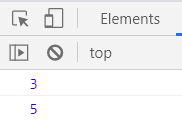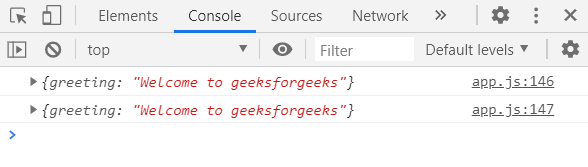Call by Value Vs Call by Reference in JavaScript

• Difficulty Level : Basic
• Last Updated : 31 Aug, 2020

Call by Value: Suppose there is a variable named “a”. Now, we store a primitive value(boolean, integer, float, etc) in the variable “a”.

Let us store an integer value in “a”, Let a=5. Now the variable “a” stores 5 and has an address location where that primitive value sits in memory.

Now, suppose we copy the value of “a” in “b” by assignment (a=b). Now, “b” points to a new location in memory, containing the same data as variable “a”.

Thus, a=b=5 but both points to separate locations in memory.

This approach is called call by value where 2 variables become the same by copying the value but in 2 separate spots in the memory.

Features of call by value:

• Function arguments are always passed by value.
• It copies the value of a variable passed in a function to a local variable.
• Both these variables occupy separate locations in memory. Thus, if changes are made in a particular variable it does not affect the other one.

Example:

JavaScript



Output: “b” was just a copy of “a”. It has its own space in memory. When we change “a” it does not have any impact on the value of “b”By value

Call by reference: Let’s say, we have an object stored in the variable “a”. The variable stores the location or the address where the object lives. Now we set b=a. Now that new variable “b” instead of pointing to a new location in the memory, points to the same location where “a” does. No new object is created, no copy is created. Both the variables point to the same object. This is like having 2 names.

This is call by reference. It behaves quite differently from by value. All objects interact by reference.

Features of By reference:

• In JavaScript, all objects interact by reference.
• If an object is stored in a variable and that variable is made equal to another variable then both of them occupy the same location in memory.
• Changes in one object variable affect the other object variable.

Example: Over here, when we set d=c, “d” points to the same location in memory where “c” does. At first, we have a name-value pair stored in “c”. Now when we change a property using “c”, it changes the property in “d” also because both point to the same object. Changes in one it affects.

Javascript



Output:By reference

Difference between call by value and call by reference:

My Personal Notes arrow_drop_up